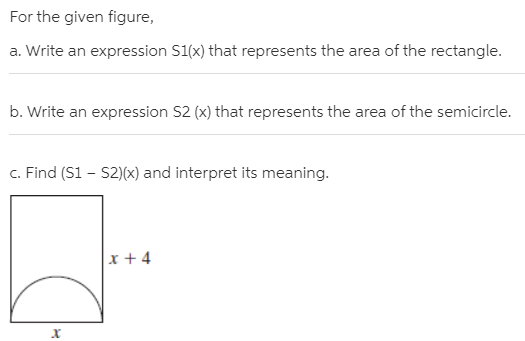# For the given figure, a. Write an expression S1(x) that represents the area of the rectangle. b. Write an expression S2 (x) that represents the area of the semicircle. c. Find (S1 – S2)(x) and interpret its meaning. x + 4

Questionhelp_outlineImage TranscriptioncloseFor the given figure, a. Write an expression S1(x) that represents the area of the rectangle. b. Write an expression S2 (x) that represents the area of the semicircle. c. Find (S1 – S2)(x) and interpret its meaning. x + 4 fullscreen